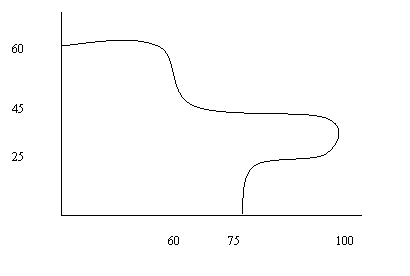# Quiz Chapter 07.02: Trapezoidal Rule

 MULTIPLE CHOICE TEST TRAPEZOIDAL RULE INTEGRATION

1. The two-segment trapezoidal rule of integration is exact for integrating at most _____ order polynomials.

2. The value of$\, \displaystyle\int_{0.2}^{2.2} xe^{x} dx$ by using the one-segment trapezoidal rule is most nearly

3. The value of$\, \displaystyle\int_{0.2}^{2.2} xe^{x} dx$ by using the three-segment trapezoidal rule is most nearly

4. The velocity of a body is given by

• $\begin{matrix} v(t) = 2t,&1 \leq t \leq 5 \\ v(t) = 5t^{2} + 3,&5< t \leq 14 \\ \end{matrix}$

where $t$ is given in seconds, and $v$ is given in m/s. Use the two-segment Trapezoidal Rule to find the distance covered by the body from $t=2$ to $t=9$ seconds.

5. The shaded area shows a plot of land available for sale. The numbers are given in meters measured from the origin. Your best estimate of the area of land in square meters is most nearly6. The following data of the velocity of a body as a function of time is given as follows

 Time (s) $0$ $15$ $18$ $22$ $24$ Velocity (m/s) $22$ $24$ $37$ $25$ $123$

The distance in meters covered by the body from $t=12$ s to $t=18$ s calculated using the Trapezoidal Rule with unequal segments is most nearly